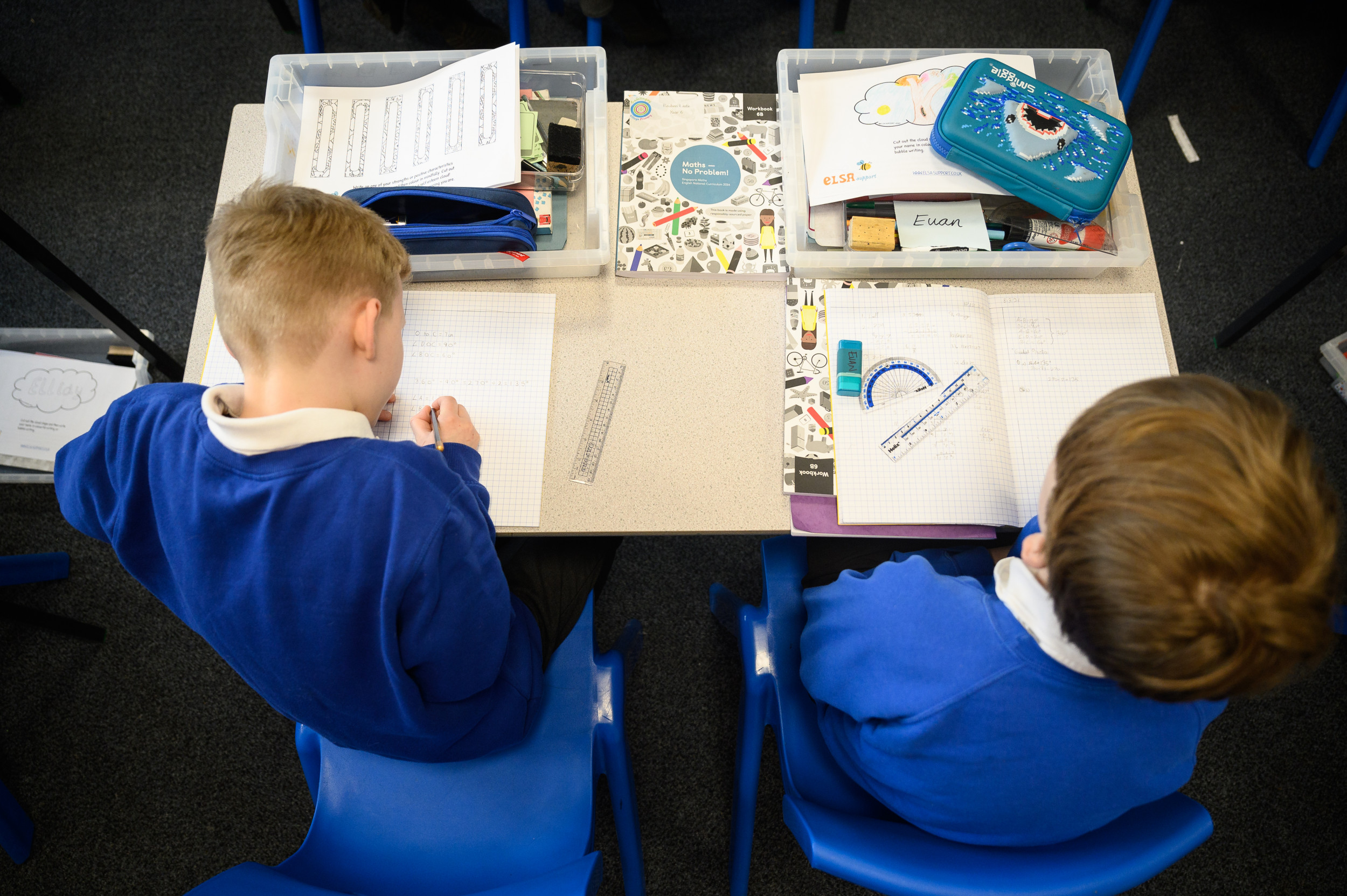# This Viral Math Problem Has the Internet in a Frenzy

Mathematics is a fundamental part of anyone's elementary and high school education. Students are taught from an early age how to add, subtract, multiply and divide numbers to solve equations.

But for many, a viral tweet is driving them crazy, with users producing different answers and vehemently disagreeing about the course of action required to solve the problem.

Twitter user @lesvity, who goes by the name The LUVITY on the platform, posted a basic algebra equation on Sunday afternoon: 6÷2(1+2). The tweet was captioned, "How can you guys get anything other than 7..."

The seemingly mundane equation quickly became a topic of controversy in the comments.

Users like @matteewyd, also known as Mattee Clownee, explained that the PEMDAS principle is required to solve the problem. As explained by Khan Academy, PEMDAS is "the order of operations ... that tells the correct sequence of steps for evaluating a math expression ... Parentheses, Exponents, Multiplication and Division, Addition and Subtraction."

With Clownee's logic, the addition of 1 and 2 needs to be solved first, as they are in a pair of parentheses. After solving and getting 3 as the answer, they multiplied it by the 2 to get 6, and dividing 6 by itself produces 1.

Thalia Reid, or user @lia_gravity, agreed with Clownee. "I [swear to God] I hate when someone brings math on this [motherf*****g] app bc y'all embarrass yourselves every damn time," she wrote. "If you still ain't get it ima pray for you."

User @ChangkyunWifey, also known as Ruki, disagreed: "The correct answer is 9." They also utilized PEMDAS to get 3 from the 1+2 in parentheses but proceeded to finish the equation going from left to right. Rather than multiplying the 2 and 3, they divided the 6 by 2 to get 3. The 3 multiplied by itself then equals 9.

"Write it into a calculator if u don't believe it," they said, before noting, "I'm really bad at math tho."

Alternatively, anonymous tweeter @sheisonfieryice agreed with The LUVITY's original answer of 7. Rather than solve 1+2 in the parentheses, they distributed the outside 2 to the 1 and 2, which via multiplication yields 2 and 4. They divided the 6 by 2 to get 3, and after adding the 4 received a final answer of 7.

User @itsmagik_, also known as Kamila Soto, explained: "the reason why people are getting different answers," focusing on the larger debate between 1 and 9, it depends on how the equation is written.

If the problem is written as if 6 were being divided by 2(1+2), the answer does yield 1. However, if it is written as the fraction 6/2 to be multiplied by 1+2, the order of distribution gives an answer of 9.

Others kept the discourse more lighthearted, with one person named Mel joking, "This is making me rethink who [I'm] friends with" after their friends produced varying results. "Schools must really suck these days," another user named Zora chimed in.

This isn't the first time in recent months Twitter has gone into a frenzy over mathematics. Back in November, Twitter users were amazed by MSNBC's Steve Kornacki's quick on-air math during the election season.A viral tweet has users debating over the math used to solve the basic algebra question. Leon Neal/Getty Images